# Procedural 2D Space Scenes in WebGL

Rye Terrell
October 22, 2016

In this post, we’re going to learn how to procedurally generate 2D space scenes like these: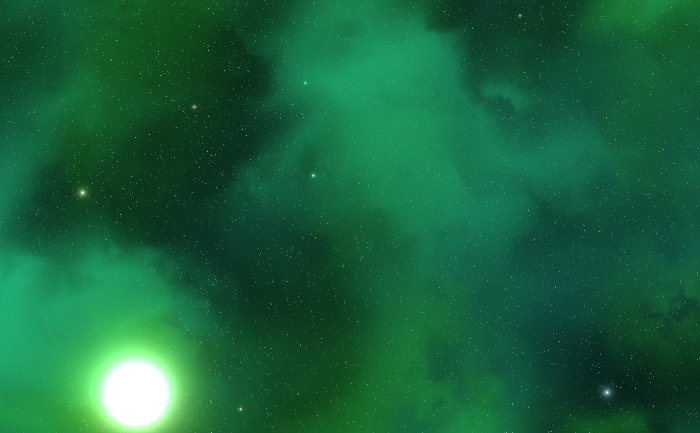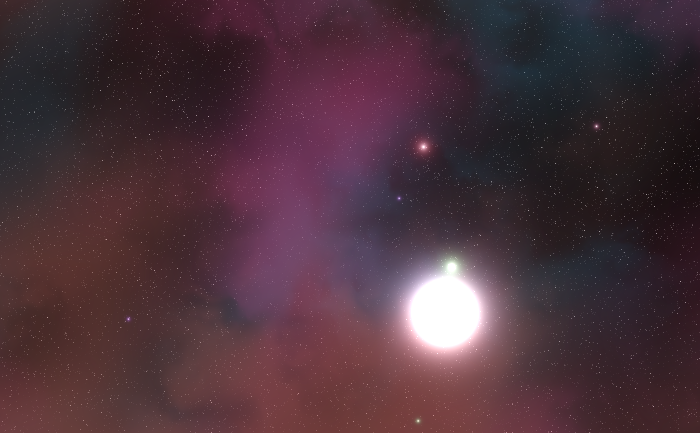Procedurally generated 2D space scenes.

You can find the space scene generator here and the source for it here. It has a completely free license, so feel free to do whatever you want with the code and the images you generate with it. Attribution is of course appreciated, but not at all required - I won’t even be mad. Go nuts!

## Overview

The space scenes we’ll be generating are composed of four parts. Point stars: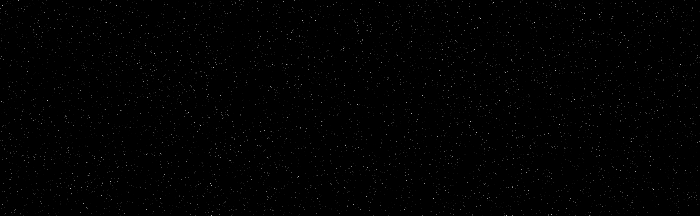Nebulae: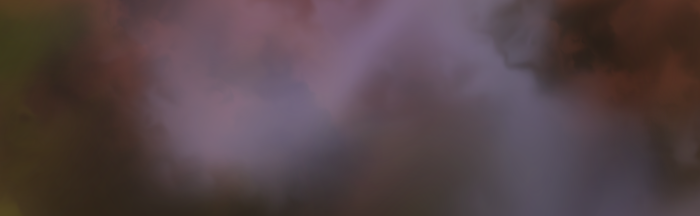Stars: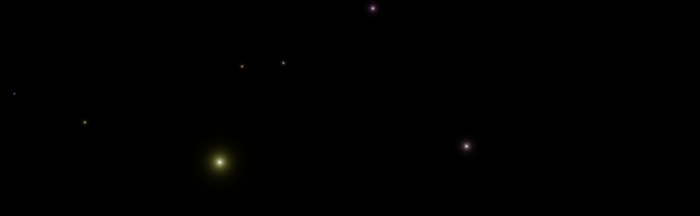And a sun: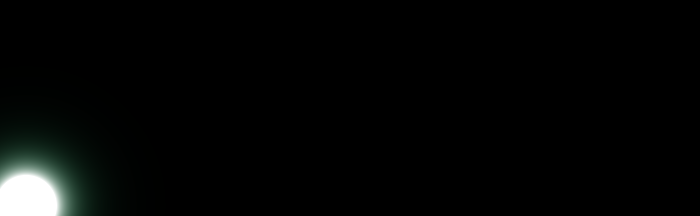These four pieces will be blended together to form a final image: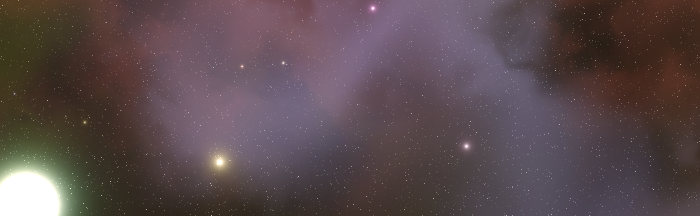### Point Stars

The point stars are single-pixel stars that we’ll use as the backdrop to our scene.

To avoid issues with pseudorandom number generators implemented in WebGL 1.0’s GLSL language, we’ll create the point star texture on the CPU. Let’s take a look at our function signature:

function generateTexture(width, height, density, brightness, prng) {


The width and height parameters are the width and height of our scene in pixels. density is a number on the range $$[0,1]$$ that describes the fraction of the total number of pixels that we’ll draw random stars for. brightness is an exponential factor that we’ll use to select each star’s brightness, and prng is a pseudorandom number generator of your choosing.

Next let’s determine the number of random point stars we’ll be generating:

let count = Math.round(width * height * density);


We’ll create a byte array to hold our texture data:

let data = new Uint8Array(width * height * 3);


Now let’s loop over the number of stars we’re going to create, count, and select a pixel with a uniform distribution from our scene:

  for (let i = 0; i < count; i++) {
let r = Math.floor(prng() * width * height);


Next we’ll randomly select a star brightness from an exponential distribution:

let c = Math.round(255 * Math.log(1 - prng()) * -brightness);


And set the color of our randomly-selected pixel to a grayscale value using the brightness:

    data[r * 3 + 0] = c;
data[r * 3 + 1] = c;
data[r * 3 + 2] = c;
}


Finally, we’ll return the byte array for our data texture:

  return data;
}


In my code, I’m using a brightness factor of around $$0.125$$ and a density factor of around $$0.05$$, which gives results like:Point stars rendered with brightness = 0.125, density = 0.05

Varying the brightness factor changes the intensity of the stars, but not their number: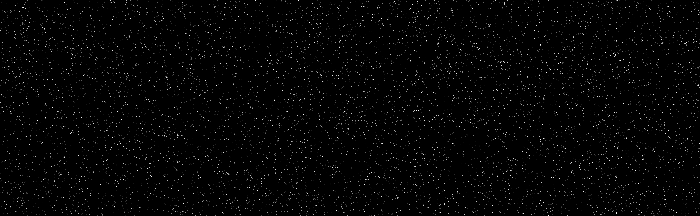Point stars rendered with brightness = 0.5, density = 0.05

While changing the density alters their count, but not their brightness: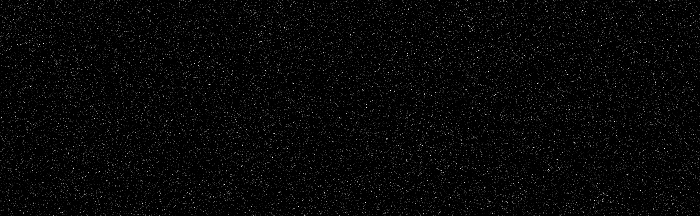Point stars rendered with brightness = 0.125, density = 0.25

You’ll need to play with these parameters to find something you find attractive.

All our vertex shaders are identical. We’re going to render a full-screen quad and interpolate the UV coordinates from $$(0, 0)$$ to $$(1, 1)$$ from the bottom left corner to the top right, respectively:

precision highp float;

attribute vec2 position, uv;

varying vec2 vUV;

void main() {
gl_Position = vec4(position, 0, 1);
vUV = uv;
}


### Nebulae

Next we’ll take a look at the fragment shader for the nebula renderer. I’ll be assuming you have a 2D Perlin Noise function. If you take a look at my code, you’ll see I’m using a homegrown 2D Perlin Noise generator that uses a texture as its source of randomness. You’re welcome to use it or another one of your choice, like this one. Whatever you use, it should be a function that takes a 2D vector and returns a floating point number on the range $$(-1, 1)$$:

float perlin_2d(vec2 p) {...}


Alright, let’s get started with our uniforms. First, source is the FBO we’ll be sampling from to blend with our current nebula. This might be just the point stars, or one or more nebulae that we’ve already blended with our point stars:

uniform sampler2D source;


Next is the RGB color of our nebula:

uniform vec3 color;


And an offset that we’ll use to shift the Perlin Noise so that the multiple nebulae we render don’t all perfectly overlap:

uniform vec2 offset;


Next is scale, which is how far into this nebula we’re going to zoom, density, which describes how opaque our nebula will be, and falloff, which governs how quickly that density varies from zero to one:

uniform float scale, density, falloff;


Finally, we’ll retrieve the interpolated UV coordinates from our vertex shader:

varying vec2 vUV;


To generate the nebulae, we’re going to be using noise on the range $$[0,1]$$, so we’ll need a function that transforms the perlin_2d noise (which is on the range $$[-1,1]$$) for us:

float normalnoise(vec2 p) {
return perlin_2d(p) * 0.5 + 0.5;
}


Next we’ll write our noise function. This will take a 2D vector and return a floating point value on the range $$[0, 1]$$:

float noise(vec2 p) {


We’ll shift our incoming vector p by offset so that our nebulae don’t all overlap perfectly:

    p += offset;


Then we’ll define a number of iterations we’re going to make, and use that value to calculate a scale that we’ll start sampling the Perlin Noise at:

    const int steps = 5;
float scale = pow(2.0, float(steps));


We’ll define a displacement value:

    float displace = 0.0;


Then we’ll iterate steps times, and each iteration we’ll calculate a new displacement based on the normalnoise at the old displacement, scaled by scale:

    for (int i = 0; i < steps; i++) {
displace = normalnoise(p * scale + displace);
scale *= 0.5;
}


Finally we’ll return the normalnoise at p (shifted by offset), plus the final displacement value we calculated:

    return normalnoise(p + displace);
}


If the above function isn’t terribly intuitive to you, don’t worry about it too much. The gist of it is that it uses Perlin Noise to sample into Perlin Noise. Once you wrap your head around that, you’ll be off to the races coming up with your own implementation - there’s a lot of room for creativity in this little function!

Now let’s take a look at the main function of our fragment shader. First we’ll sample our source FBO to see what color the pixel is before we alter it:

void main() {
vec3 s = texture2D(source, vUV).rgb;


Then we’ll calculate the nebula’s noise value at our current pixel location, scaled by our uniform scale:

  float n = noise(gl_FragCoord.xy * scale * 1.0);


Next we adjust that noise value, bumping it up by density and then raising it to falloff:

  n = pow(n + density, falloff);


Finally we mix our incoming color with our nebula color by the density we calculated, n, and set our pixel to that mixed color:

  gl_FragColor = vec4(mix(s, color, n), 1);
}


Now that we have our vertex and fragment shaders, let’s take a look at using them with the WebGL API. To this end, I’ll be leveraging the fantastic regl library, which, if you haven’t checked out - you really should!

Let’s create a NebulaRenderer regl command:

let NebulaRenderer = regl({


It’ll take the vertex and fragment shaders we just created:

  vert: vertexShader,


And we’ll pass it position and UV coordinates for our full-screen quad:

  attributes: {
position: regl.buffer([-1, -1, 1, -1, 1, 1, -1, -1, 1, 1, -1, 1]),
uv: regl.buffer([0, 0, 1, 0, 1, 1, 0, 0, 1, 1, 0, 1])
},


We’ll pass in the uniforms we need:

  uniforms: {
source: regl.prop('source'),
offset: regl.prop('offset'),
scale: regl.prop('scale'),
falloff: regl.prop('falloff'),
color: regl.prop('color'),
density: regl.prop('density'),
},


A framebuffer that we’ll be rendering to:

  framebuffer: regl.prop('destination'),


A viewport that covers the entire scene:

  viewport: regl.prop('viewport'),


And finally indicate the number of vertices we’ll be rendering:

  count: 6
});


That’s it! Now when we call NebulaRenderer, we supply the uniforms describing our nebula and the framebuffer we want to render to, and it renders it for us. Nice and clean.

In my code, I use values on the range $$(0, 0.2)$$ for density and $$(3, 5)$$ for falloff. Here’s some samples in and out of that range: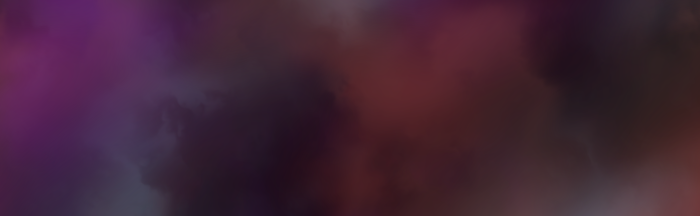Nebulae rendered with density = 0.1 and falloff = 4.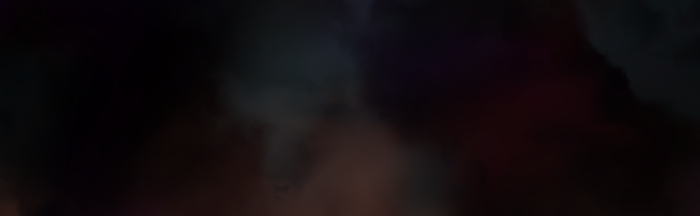Nebulae rendered with density = 0.1 and falloff = 8.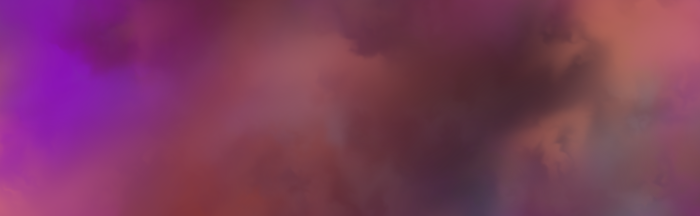Nebulae rendered with density = 0.25 and falloff = 4.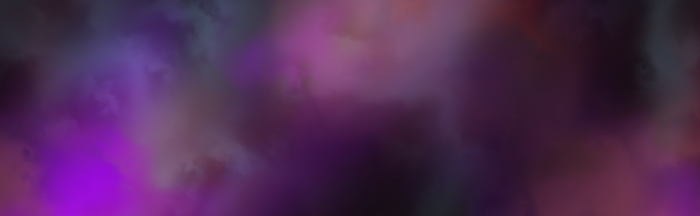Nebulae rendered with density = 0.25 and falloff = 8.

### Sun & Stars

The sun and stars (the larger, non-point stars) are both rendered with the same code, but with different parameters - namely that the sun is larger! I’ll use “star” to reference both.

Let’s take a look at the fragment shader. We’ll start with the uniforms again. First, the source framebuffer that we’ll be blending with:

uniform sampler2D source;


Then the RGB values for the core of the star and the halo of the star:

uniform vec3 coreColor, haloColor;


Then the 2D vectors representing the location of the star and the resolution of our scene, center and resolution, respectively:

uniform vec2 center, resolution;


Next, coreRadius, which is the radius of our star’s primary mass, haloFalloff, which governs how far out the halo of our star reaches, and scale, which is used to describe how the radius maps to the size of our scene:

uniform float coreRadius, haloFalloff, scale;


Finally, we’ll bring in the interpolated UV coordinates from our trusty vertex shader:

varying vec2 vUV;


Alright, let’s take a look at rendering a star. First, we’ll sample the color of the incoming texture that we’re blending with:

void main() {
vec3 s = texture2D(source, vUV).rgb;


Then we’ll calculate the distance from our current pixel to the center of our star, and modify it by scale:

  float d = length(gl_FragCoord.xy - center * resolution) / scale;


If that distance is less than or equal to our coreRadius, we want it to be completely opaque, not blended:

  if (d <= coreRadius) {
gl_FragColor = vec4(coreColor, 1);
return;
}


Otherwise, let’s calculate a blending factor based on an exponential function modified by haloFalloff:

  float e = 1.0 - exp(-(d - coreRadius) * haloFalloff);


Then we’ll calculate a mixed value, rgb, between our core color and halo color:

  vec3 rgb = mix(coreColor, haloColor, e);


And then mix that value with zero to make it fade to nothing:

  rgb = mix(rgb, vec3(0,0,0), e);


Finally, we’ll perform an additive blending operation with our source color and set the fragment to the result:

  gl_FragColor = vec4(rgb + s, 1);
}


Now we can take a look at our StarRenderer regl command, which probably doesn’t need any explanation after seeing NebulaRenderer above:

let StarRenderer = regl({
attributes: {
position: regl.buffer([-1, -1, 1, -1, 1, 1, -1, -1, 1, 1, -1, 1]),
uv: regl.buffer([0, 0, 1, 0, 1, 1, 0, 0, 1, 1, 0, 1]),
},
uniforms: {
center: regl.prop("center"),
coreColor: regl.prop("coreColor"),
haloColor: regl.prop("haloColor"),
haloFalloff: regl.prop("haloFalloff"),
resolution: regl.prop("resolution"),
scale: regl.prop("scale"),
source: regl.prop("source"),
},
framebuffer: regl.prop("destination"),
viewport: regl.prop("viewport"),
count: 6,
});


### Putting it all together

#### The CopyRenderer

We’ll use a regl command that performs a very simple copy operation using a source and destination framebuffer. The only thing here that is perhaps not obvious is that if the destination, framebuffer, is undefined, it will render to the screen (the associated DOM Canvas element) instead of another framebuffer. Here it is:

let CopyRenderer = regl({
frag: 
precision highp float;
uniform sampler2D source;
varying vec2 vUV;
void main() {
gl_FragColor = texture2D(source, vUV);
}
,
attributes: {
position: regl.buffer([-1, -1, 1, -1, 1, 1, -1, -1, 1, 1, -1, 1]),
uv: regl.buffer([0, 0, 1, 0, 1, 1, 0, 0, 1, 1, 0, 1]),
},
uniforms: {
source: regl.prop("source"),
},
framebuffer: regl.prop("destination"),
viewport: regl.prop("viewport"),
count: 6,
});


#### Ping-Ponging

Ping-ponging is a technique wherein you alternate the source and destination framebuffers as you run multiple GLSL programs so that the output of one execution is the input of the next. While you could create $$N$$ framebuffers to accomplish the same thing, this reduces resource usage, which can be particularly important if you’re executing more than a handful of times to get your final result.

We’ll need to create some framebuffers for ping-ponging:

let ping = regl.framebuffer({
color: regl.texture(),
depth: false,
stencil: false,
depthStencil: false,
});
let pong = regl.framebuffer({
color: regl.texture(),
depth: false,
stencil: false,
depthStencil: false,
});


We’ll also write a convenience function to handle most of the swapping stuff for us. Let’s take a look at that. First, the function signature. We’ll take an initial framebuffer, which will be our first source framebuffer. Then alpha and beta framebuffers, which we’ll alternate between as source and destination framebuffers. We’ll alternate count times, and we’ll run a callback render function func each time:

function pingPong(initial, alpha, beta, count, func) {


If count is zero, our output should simply be initial, so return that:

if (count === 0) return initial;


We’ll use alpha as our first destination framebuffer, so if initial and alpha are the same framebuffer, we’ll swap alpha and beta. We do this because we cannot both read from and render to the same framebuffer at the same time.

if (initial === alpha) {
alpha = beta;
beta = initial;
}


Next we’ll call func, using initial as the source and alpha as the destination.

func(initial, alpha);


We need to keep track of the number of times we’ve called func, which is now one:

let i = 1;


If count is one, we only want to render once, which we’ve already done, so let’s bail and return alpha.

if (i === count) return alpha;


Otherwise, let’s loop:

  while (true) {


Call func with alpha as our source and beta as our destination. Increment our count, and if we’ve hit our target, return beta.

func(alpha, beta);
i++;
if (i === count) return beta;


Do the same thing, but this time with beta as the source and alpha as the destination:

func(beta, alpha);
i++;
if (i === count) return alpha;


At some point one of those two cases will be true and we’ll have returned the destination framebuffer.

  }
}


#### Render & blend

First we’ll generate our backdrop - the point stars. We’ll call our function, using Math.random as the pseudorandom number generator:

let data = pointStars.generateTexture(width, height, 0.05, 0.125, Math.random);


Then we’ll create a regl texture object with it:

let pointStarTexture = regl.texture({
format: "rgb",
width: width,
height: height,
wrapS: "clamp",
wrapT: "clamp",
data: data,
});


And finally copy that to our ping framebuffer:

CopyRenderer({
source: pointStarTexture,
destination: ping,
viewport: viewport
});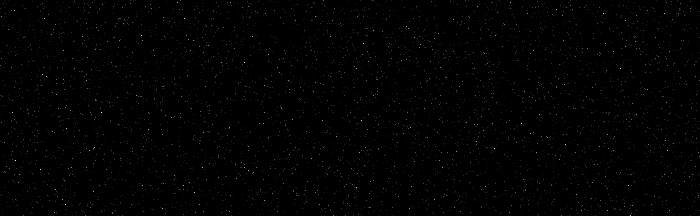After rendering the point stars.

Next we’ll render & blend nebulae on top of the point stars. First, we’ll figure out how many to render:

let nebulaCount = Math.round(rand.random() * 4 + 1);


Then we’ll use our pingPong function to render the nebulae. initial will be the ping framebuffer (which is the output framebuffer of our point star rendering), alpha and beta are ping and pong, count is nebulaCount, and our callback render function func is a wrapper around NebulaRenderer that handles the source and destination parameters provided by the pingPong function:

let nebulaOut = pingPong(ping, ping, pong, nebulaCount, (source, destination) => {
NebulaRenderer({
source: source,
destination: destination,
offset: [rand.random() * 100, rand.random() * 100],
scale: (rand.random() * 2 + 1) / scale,
color: [rand.random(), rand.random(), rand.random()],
density: rand.random() * 0.2,
falloff: rand.random() * 2.0 + 3.0,
width: width,
height: height,
viewport: viewport,
});
});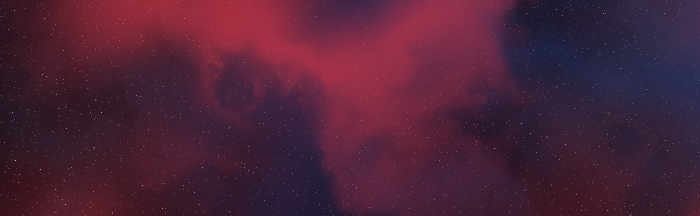After blending the nebulae.

Next we’ll render the stars in a similar fashion, using nebulaOut from above as initial:

let starCount = Math.round(rand.random() * 8 + 1);
let starOut = pingPong(nebulaOut, ping, pong, starCount, (source, destination) => {
StarRenderer({
center: [rand.random(), rand.random()],
coreColor: [1, 1, 1],
haloColor: [rand.random(), rand.random(), rand.random()],
haloFalloff: rand.random() * 1024 + 32,
resolution: [width, height],
scale: scale,
source: source,
destination: destination,
viewport: viewport,
});
});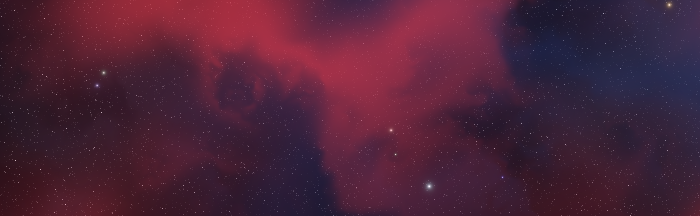After blending the stars.

And finally we’ll render the sun, setting destination to undefined to make it render to the screen, and using starOut as our source framebuffer:

StarRenderer({
center: [rand.random(), rand.random()],
coreRadius: rand.random() * 0.025 + 0.025,
coreColor: [1, 1, 1],
haloColor: [rand.random(), rand.random(), rand.random()],
haloFalloff: rand.random() * 32 + 32,
resolution: [width, height],
scale: scale,
source: starOut,
destination: undefined,
viewport: viewport,
});Our final scene after blending the sun.

### All done!

Congratulations for making it this far! If you’ve got WebGL running in your browser (most people do these days), you should be able to play with the scene generator below. Have fun!